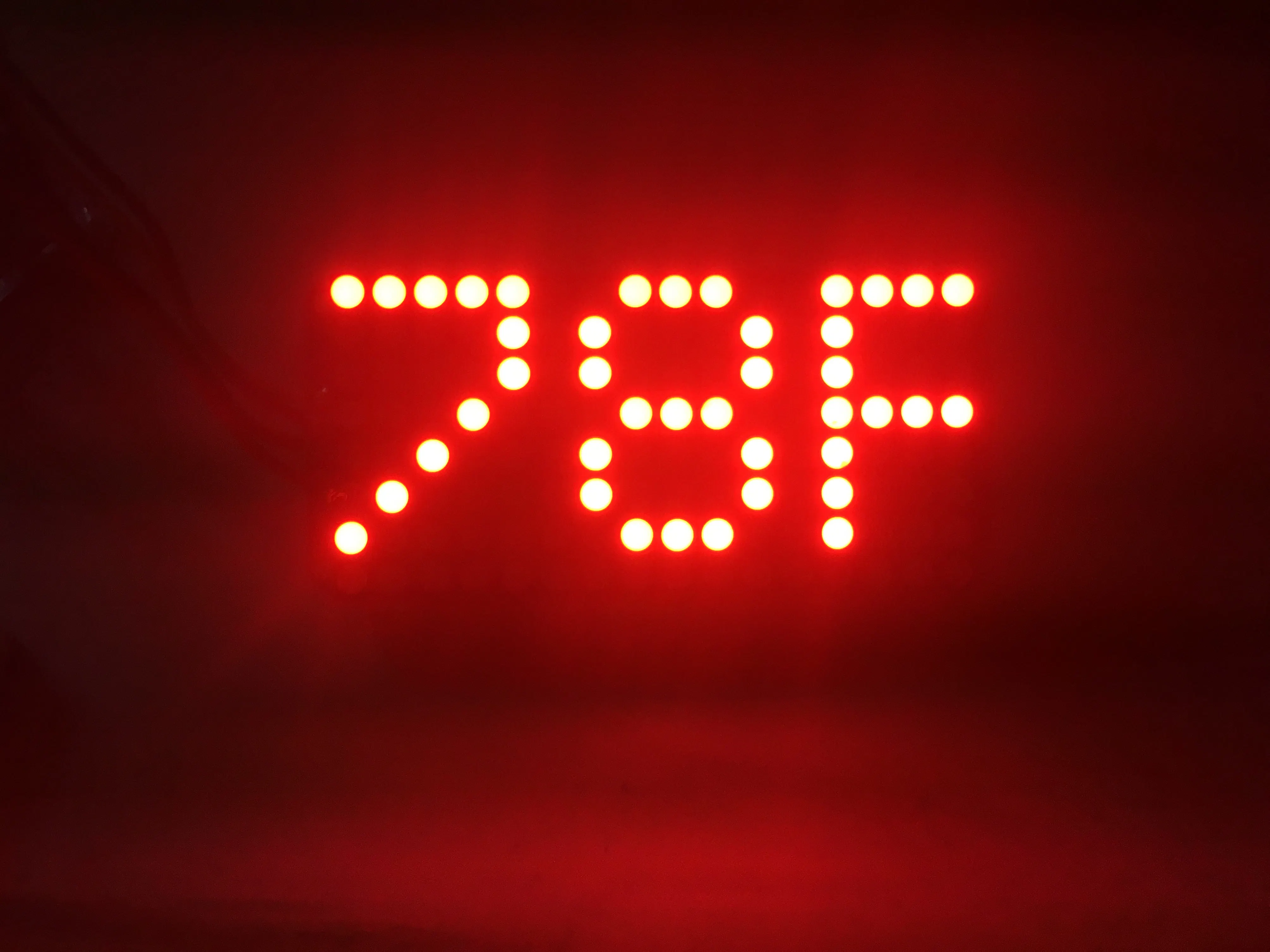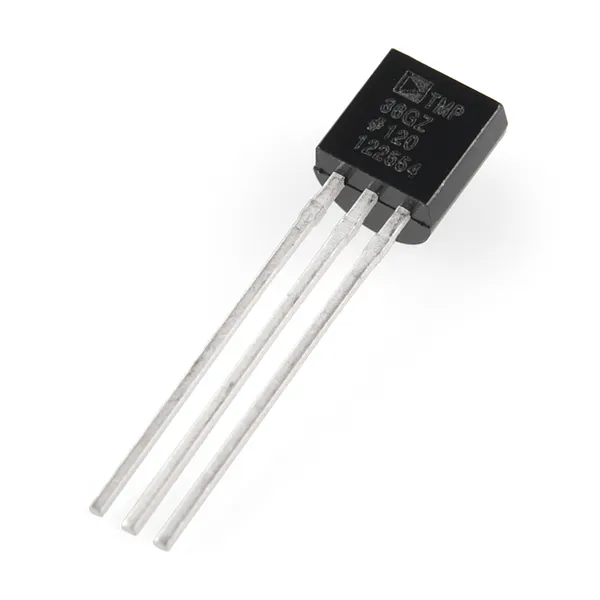Published

# Periodic Temperature Reporter

This small and attractive device periodically displays an attention-catching animation followed by the current temperature in C° and F°.

IntermediateFull instructions provided1,931## Things used in this project

### Hardware components×1Arduino UNO & Genuino UNO Although any similar boards should work Also, it is assumed that one has a way of downloading sketches onto the board
×1
 Adafruit 0.8" 8x16 LED Matrix FeatherWing Display Multiple colors available
×1Temperature Sensor
×1Jumper wires (generic)
×7

### Software apps and online servicesArduino IDE

## Schematics

### Temperature Reporter Design

(picture of schematics can also be seen above the story)
The design shows all the wires that are used and where they go. In this project I used and Ada Fruit 16/8 LED Matrix that did not show up in the program so I improvised by drawing wire to notes that had written the place where they needed to be connected on the matrix.

## Code

### TemperatureReporter.ino

Arduino
Here there are seven different classes that work together to create the effect.
```#include <Adafruit_GFX.h>
#include "variables.h"

//TMP36 Pin Variables
int sensorPin = 0;
void setup() {
Serial.begin(9600); // matching response speed between the arduino and the computer
Serial.println("minute by minute temperature sensor");
matrix.begin(0x70);  // passes in the address
}

void loop() {
matrix.setTextSize(1); // sets text size
matrix.setTextWrap(false);  // makes the text scroll nicely
matrix.setTextColor(LED_ON);
matrix.setRotation(1); // defines the texts orientation

sense();
left();
delay(60000);
sense();
top();
delay(60000);
sense();
right();
delay(60000);
sense();
bottom();
delay(60000);
}
```

### sense.ino

Arduino
```void sense() {

// converts the reading to volts, for 3.3v arduino use 3.3
voltage = reading * 5.0 / 1024.0;

// prints out the voltage
Serial.print(voltage); Serial.println(" volts");

// converts to Celcius
temperatureC = (voltage - 0.5) * 100 ;
Serial.print(temperatureC); Serial.println(" degrees C"); // prints Celcius

// converts to Fahrenheit
temperatureF = (temperatureC * 9.0 / 5.0) + 32.0;
Serial.print(temperatureF); Serial.println(" degrees F"); // prints Fahrenheit
}
```

### top.ino

Arduino
```void top() {
// scrolls from top to the bottom
a = -7;
for (x=0; x<=14; x++) {
matrix.clear();
matrix.setCursor(0,a);
matrix.print("!!!");
matrix.writeDisplay();
matrix.setCursor(-1,a);
matrix.print("!!!");
matrix.writeDisplay();
delay(100);
a += 1;
b += 1;
}

for (x=-8; x<=0; x++) {
matrix.clear();
matrix.setCursor(0,x);
matrix.print(temperatureF);
delay(50);
matrix.print("F ");
delay(50);
matrix.writeDisplay();
delay(50);
}
delay(1000);

for (x=-8; x<=0; x++) {
matrix.clear();
matrix.setCursor(0,x);
matrix.print(temperatureC);
delay(30);
matrix.print("C");
delay(30);
matrix.writeDisplay();
delay(30);
}
delay(1000);
matrix.clear();
matrix.writeDisplay();
}
```

### bottom.ino

Arduino
```void bottom() {
// scrolls from bottom to the top
a = 7;
b = 8;
for (x=0; x<=11; x++) {
matrix.clear();
matrix.setCursor(0,a);
matrix.print("^^^");
matrix.writeDisplay();
matrix.setCursor(0,b);
matrix.print("^^^");
matrix.writeDisplay();
delay(100);
a -= 1;
b -= 1;
}

for (x=8; x>=0; x--) {
matrix.clear();
matrix.setCursor(0,x);
matrix.print(temperatureF);
delay(50);
matrix.print("F ");
delay(50);
matrix.writeDisplay();
delay(50);
}
delay(1000);

for (x=8; x>=0; x--) {
matrix.clear();
matrix.setCursor(0,x);
matrix.print(temperatureC);
delay(30);
matrix.print("C");
delay(30);
matrix.writeDisplay();
delay(30);
}
delay(1000);
matrix.clear();
matrix.writeDisplay();
}
```

### left.ino

Arduino
```void left() {
// scrolls from left to right
a = -23;
b = -24;
for (x=-20; x<=0; x++) {
matrix.clear();
matrix.setCursor(a,0);
matrix.print(">>>>");
matrix.writeDisplay();
matrix.setCursor(b,0);
matrix.print(">>>>");
matrix.writeDisplay();
delay(100);
a += 2;
b += 2;
}

for (x=-16; x<=0; x++) {
matrix.clear();
matrix.setCursor(x,0);
matrix.print(temperatureF);
delay(30);
matrix.print("F ");
delay(30);
matrix.writeDisplay();
delay(30);
}
delay(1000);

for (x=-16; x<=0; x++) {
matrix.clear();
matrix.setCursor(x,0);
matrix.print(temperatureC);
delay(30);
matrix.print("C");
delay(30);
matrix.writeDisplay();
delay(30);
}
delay(1000);
matrix.clear();
matrix.writeDisplay();
}
```

### right.ino

Arduino
```void right() {
// scrolls from right to left
a = 17;
b = 18;
for (x=0; x<=21; x++) {
matrix.clear();
matrix.setCursor(a,0);
matrix.print("<<<<");
matrix.writeDisplay();
matrix.setCursor(b,0);
matrix.print("<<<<");
matrix.writeDisplay();
delay(100);
a -= 2;
b -= 2;
}

for (x=16; x>=0; x--) {
matrix.clear();
matrix.setCursor(x,0);
matrix.print(temperatureF);
delay(30);
matrix.print("F ");
delay(30);
matrix.writeDisplay();
delay(30);
}
delay(1000);

for (x=16; x>=0; x--) {
matrix.clear();
matrix.setCursor(x,0);
matrix.print(temperatureC);
delay(30);
matrix.print("C");
delay(30);
matrix.writeDisplay();
delay(30);
}
delay(1000);
matrix.clear();
matrix.writeDisplay();
}
```

### variables.h

Arduino
```  int a;
int b;
float voltage;